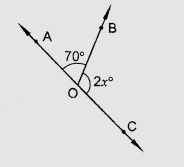"
">

# In the figure, AOC is a line, find $x$."

Given:

AOC is a line.

To do:

We have to find the value of $x$.

Solution:

We know that,

Sum of the angles on a straight line is $180^o$.

Therefore,

From the figure,

$\angle AOB + \angle COB = 180^o$

$70^o + 2x^o = 180^o$

$2x = 180^o - 70^o$

$2x= 110^o$

$x = 55^o$

The value of $x$ is $55^o$.

Updated on: 10-Oct-2022

23 Views